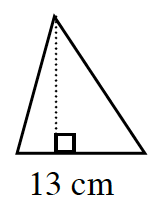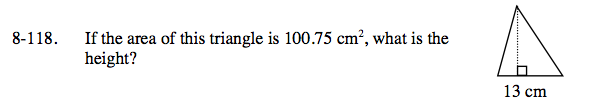Home > CC1 > Chapter 8 > Lesson 8.3.3 > Problem8-118

8-118.

If the area of this triangle is 100.75cm2, what is the height? Homework Help ✎$\text{Area of a triangle} =\frac{1}{2} (\text{base})(\text{height})$

Substitute all the values that are known.

$\frac{1}{2}(13)h=100.75$

Now solve for h.